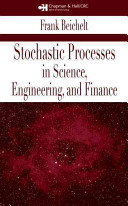### Stochastic Processes

Data is displayed for academic year: 2023./2024.

#### Course Description

In the introductory part the conditional probability, conditional expectation and technique conditioning by random variables will be studied. Then the analysis of the series of random variables and random walk will be given. The central part is the study of Markov chains and processes, and models based on these processes. The properties of the most important processes, Poisson and Wiener processes will be studied in details.

#### Study Programmes

[FER3-EN] Computing - study
Elective Courses (6. semester)
[FER3-EN] Electrical Engineering and Information Technology - study
Elective Courses (6. semester)

#### Learning Outcomes

1. Understand the basic principles of stochastic processes
2. Learn to distinguish between stochastic processes according to their properties.
3. Understand the characteristic lack of memory in different cases
4. To interpret the behavior of the process in accordance with the theoretical laws.
5. Determine the probability of prominent events related to stochastic processes.
6. Learn to model simple problems using stochastic techniques.
7. Apply stochastic techniques in the analysis of various systems.

#### Forms of Teaching

Lectures

4 hours per week

Partial e-learning

Online materials.

Independent assignments

Each student must solve some problems on his own.

Continuous Assessment Exam
Quizzes 0 % 20 % 0 % 0 %
Seminar/Project 0 % 15 % 0 % 0 %
Mid Term Exam: Written 0 % 40 % 0 %
Final Exam: Written 0 % 40 %
Exam: Written 0 % 100 %

#### Week by Week Schedule

1. Conditional distribution and expectation. Conditioning on a random variable. Conditioning on a sigma-field.
2. Sums of independent random variables. Stopping times Wald identities. Generating functions.
3. Random walks. Probability of ruin. Recurrent events.
4. Stochastic processes. Markov process. Foundations and examples. Construction of Markov chains. Transition probabilities and the Chapman-Kolmogorov equation. Stopping times and strong Markov property. Absorbing states. Transient and recurrent states. Branching processes.
5. Limit theorems and stationary distributions. State classification. Ergodic theorems. Finite-dimensional distributions of processes. Moments, correlation and covariation functions. Classes of processes: Markov, homogenous Markov, weak/strong stationary, independent increment processes. Transition and density matrix and Chapman-Kolmogorov equation for Markov processes.
6. Poisson process. Homogeneous Poisson processes. Memoryless property.
7. Poisson processes and uniform, exponential and binomial distributions, Nonhomogeneous Poisson processes. Mixed and Compound Poisson Processes. Poisson arrivals.
8. Midterm exam
9. Continuous-time Markov process. Basic concepts and examples. Transition probabilities and rates. Birth and death processes. Kolmogorov differential equations. Stationary state probabilities. Ergodic theorems.
10. Renewal processes. Renewal Functions. Excess life, current life and total life. Strong laws of large numbers. Recurrence times. Terminating renewal processes. Stationary renewal processes. Alternating Renewal Processes.
11. Queueing models. Basic concepts. The Erlang model, M/M/1 and M/M/c queue.
12. Loss systems. M/M systems. Waiting systems. M/G and G/M models. Network of queueing systems
13. Introduction. Properties of Brownian motion. Multidimensional and conditional distributions. First passage times.
14. Brownian motion. Transformations of the Brownian motion. Brownian motion with drift. White noise. Diffusion processes.
15. Final exam

#### Literature

(.), Neven Elezović, Stohastički procesi,Frank Beichelt (2006.), Stochastic Processes in Science, Engineering and Finance, Chapman & Hall/CRC

#### General

ID 223385
Summer semester
5 ECTS
L1 English Level
L3 e-Learning
60 Lectures
0 Seminar
0 Exercises
0 Laboratory exercises
0 Project laboratory